# Introduction and models used

In the real problem, there is the presence of three phases : air, oil and water. The study of multiphase flow is often complex. It's why, tackling directly the three phase-flow resolution could be very difficult and could lead to a misunderstanding of the simplest principles of the problem. Then  we decided, first of all to begin our study with simple cases and studying them for different parameters combinations.

In the one phase flow case we consider that there is only one fluid presents in the whole domain of resolution and we resolve the simple conservation equation in which Darcy law is injected.

We used the following equations :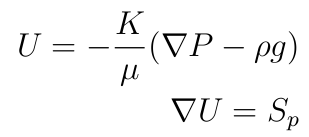where :

$U$ :  the velocity field

$P$ : the pressure

$S_p$ :  the source term

$K$ : the permeability

$g$ : the gravity

$\rho$ : the density

$\mu$ : the dynamic viscosity

In some studies the gravity will be neglected.

To resolve the conservation equation in which Darcy law is injected  we developed a solver that we called a Darcy solver or darcyFoam by modifying the existing laplacianFoam solver in OpenFoam solver directory. Then we modified it in order to compute the pressure field and having Darcy velocity.

After developing this simple Darcy solver, we added a source term that corresponds to the rate flow in the extractor well.

The second step was the definition of the permeability, no more as a simple scalar but as  a scalar field or as a tensor.

Finally we added to our solver the gravity, which  needed a real understanding of the gravity influence on boundaries conditions of the domain of study. Indeed the gravity introduction leads to a new boundary condition on the pressure gradient on the bottom of the domain and the inversion of the permeability tensor which is complex to program on OpenFoam. The boundary condition on the bottom will be expressed by :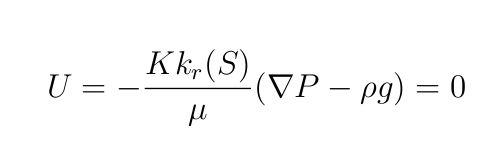On OpenFoam  we used flux through cells surface to set this boundary condition, then the condition of zero velocity on the domain bottom is transformed to a condition of zero flux.

The flux is expressed by :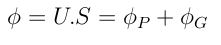S is the cell surface vector, where :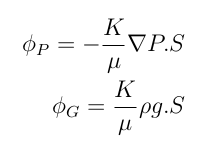Moreover $U=0$ leads to $\phi=0$,  then :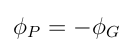Finally we have the following boundary condition on the pressure gradient :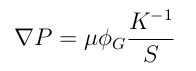To avoid having a matrix inversion, the permeability K was defined as a simple scalar or as a field of scalar as a first approach.

When the permeability is defined as a scalar or as a scalar field have :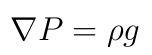In this parts we will study the influence of the anisotropy, the gravity and the flow rate on the flow to try different parameters and to study the stability of the solver.

In all the studies of this part, the fluid has the following physical properties :

$\rho=1000 \ Kg/m^3$

$\mu=6,4 \ 10^{-4} \ Kg/(m.s)$

Those properties are almost the properties of petrol.

Moreover the flow rate  called qwell will be fixed to :

$qwell=1 \ m^3/s$.